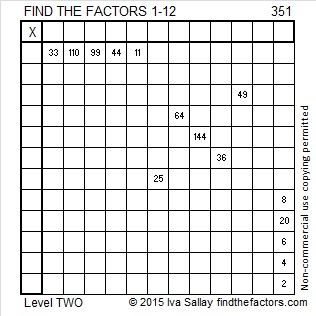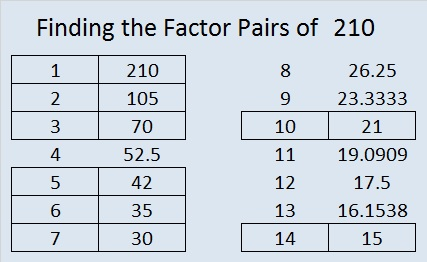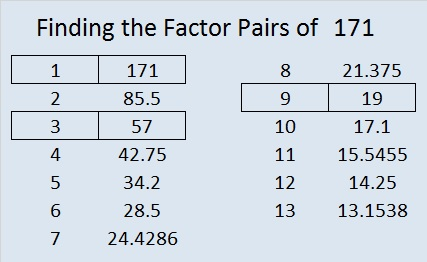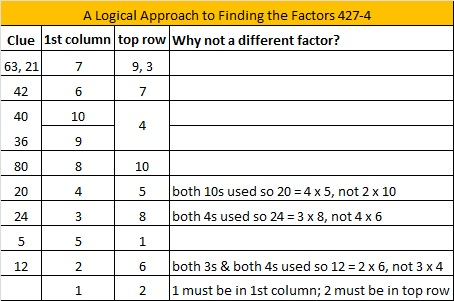# 351 is a Triangular Number

351 is the 26th triangular number. The only triangular numbers that are NOT composite numbers are 1 and 3. All other triangular numbers are composite numbers because they can be written as the product of two consecutive numbers divided by 2. For example, 351 can be written like this:

• 1 + 2 + 3 + . . . + 24 + 25 + 26 = (26 x 27)/2 = 351

Also, 351 is divisible by 3 because 1, 3, 5 are three consecutive odd numbers. Since 3 is the middle number in that list, 351 is also divisible by 9. Scroll down past the puzzle to see the rest of 351’s factors.Print the puzzles or type the factors on this excel file: 12 Factors 2015-01-12

• 351 is a composite number.
• Prime factorization: 351 = 3 x 3 x 3 x 13, which can be written (3^3) x 13
• The exponents in the prime factorization are 3 and 1. Adding one to each and multiplying we get (3 + 1)(1 + 1) = 4 x 2 = 8. Therefore 351 has exactly 8 factors.
• Factors of 351: 1, 3, 9, 13, 27, 39, 117, 351
• Factor pairs: 351 = 1 x 351, 3 x 117, 9 x 39, or 13 x 27
• Taking the factor pair with the largest square number factor, we get √351 = (√9)(√39) = 3√39 ≈ 18.735# 210 and Level 4

• 210 is a composite number and a triangular number.
• Prime factorization: 210 = 2 x 3 x 5 x 7, so 210 is the product of the first four prime numbers!
• The exponents in the prime factorization are 1, 1, 1, and 1. Adding one to each and multiplying we get (1 + 1)(1 + 1)(1 + 1)(1 + 1) = 2 x 2 x 2 x 2 = 16. Therefore 210 has 16 factors.
• Factors of 210: 1, 2, 3, 5, 6, 7, 10, 14, 15, 21, 30, 35, 42, 70, 105, 210
• Factor pairs: 210 = 1 x 210, 2 x 105, 3 x 70, 5 x 42, 6 x 35, 7 x 30, 10 x 21, or 14 x 15
• Since 210 has no square factors, √210 cannot be simplified. √210 ≈ 14.491Because 20 + 1 = 21 and (20/2) x 21 = 210, we know that 1 + 2 + 3 + 4 + 5 + 6 + 7 + 8 + 9 + 10 + 11 + 12 + 13 + 14 + 15 + 16 + 17 + 18 + 19 + 20 = 210, thus it is the 20th triangular number.

Excel file of puzzles and previous week’s factor solutions: 10 Factors 2014-08-18# 171 and Level 4

 171 is a composite number. Factor pairs: 171 = 1 x 171, 3 x 57, or 9 x 19 Factors of 171: 1, 3, 9, 19, 57, 171 Prime factorization: 170 = 3 x 3 x 19, which can be written 170 = (3^2) x 19171 = 1 + 2 + 3 + 4 + 5 + 6 + 7 + 8 + 9 + 10 + 11 + 12 + 13 + 14 + 15 + 16 + 17 + 18 and is therefore a triangular number.

The fact that 171 is a triangular number is easy to recognize because 170 = 9 x 19 = (18/2) x 19. Any integer resulting from n(n + 1)/2 is a triangular number.Excel file of puzzles and previous week’s factor solutions: 10 Factors 2014-07-07